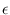Next: Basic Data Types Up: Number Types and Linear Previous: Real-Valued Matrices ( real_matrix   Contents   Index

Subsections

# Numerical Analysis Functions ( numerical_analysis )

We collect some functions of numerical analysis. The algorithms in this section are not the best known and are not recommended for serious use. We refer the reader to the book Numerical Recipes in C: The Art of Scientific Computing'' by B.P. Flannery, W.H. Press, S.A. Teukolsky, and W.T. Vetterling, Cambridge University Press for better algorithms.

The functions in this section become available by including numerical_analysis.h.

## Minima and Maxima

 double minimize_function(double (*f)(double), double& xmin, double tol = 1.0e-10) finds a local minimum of the function f of one argument. The minimizing argument is returned in xmin and the minimal function value is returned as the result of the function. xmin is determined with tolerance tol, i.e., the true value of the minimizing argument is contained in the interval [xmin(1 -), xmin(1 +)], where= max(1, xmin)*tol. Please do not choose tol smaller than 10-15. Precondition: If +or -is a local minimum of f, then the call of minimize_function may not terminate. The algorithm is implemented as follows: First three arguments are determined such that a < b < c (or a > b > c) and f (a) > = f (b) < = f (c), i.e., a and c bracket a minimum. The interval is found by first taking two arbitrary arguments and comparing their function values. The argument with the larger function value is taken as a. Then steps of larger and larger size starting at b are taken until a function value larger than f (b) is found. Once the bracketing interval is found, golden-ratio search is applied to it. template double minimize_function(const F& f, double& xmin, double tol = 1.0e-10) a more flexible version of the above. It is assumed that class F offers the operator double operator()(double x). This operator is taken as the function f.

## Integration

 double integrate_function(double (*f)(double), double l, double r, double delta = 1.0e-2) Computes the integral of f in the interval [l, r] by forming the sum delta*f (l + i*delta), where K = (r - l )/delta. Precondition l < = r and delta > 0. template double integrate_function(const F& f, double l, double r, double delta=1.0e-2) a more flexible version of the above. It is assumed that class F offers the operator double operator()(double x). This operator is taken as the function f.

## Useful Numerical Functions

 double binary_entropy(double x) returns the binary entropy of x, i.e., - x*log x - (1 - x)*log(1 - x). Precondition 0 < = x < = 1.

## Root Finding

 double zero_of_function(double (*f)(double), double l, double r, double tol = 1.0e-10) returns a zero x of f. We have either | f (x)| < = 10-10 or there is an interval [x0, x1] containing x such that f (x0)*f (x1) < = 0 and x1 - x0 < = tol*max(1,| x1| + | x1|). Precondition l < = r and f (l )*f (r) < = 0. template double zero_of_function(const F& f, double l, double r, double tol = 1.0e-10) a more flexible version of the above. It is assumed that class F offers the operator double operator()(double x). This operator is taken as the function f.Next: Basic Data Types Up: Number Types and Linear Previous: Real-Valued Matrices ( real_matrix   Contents   Index